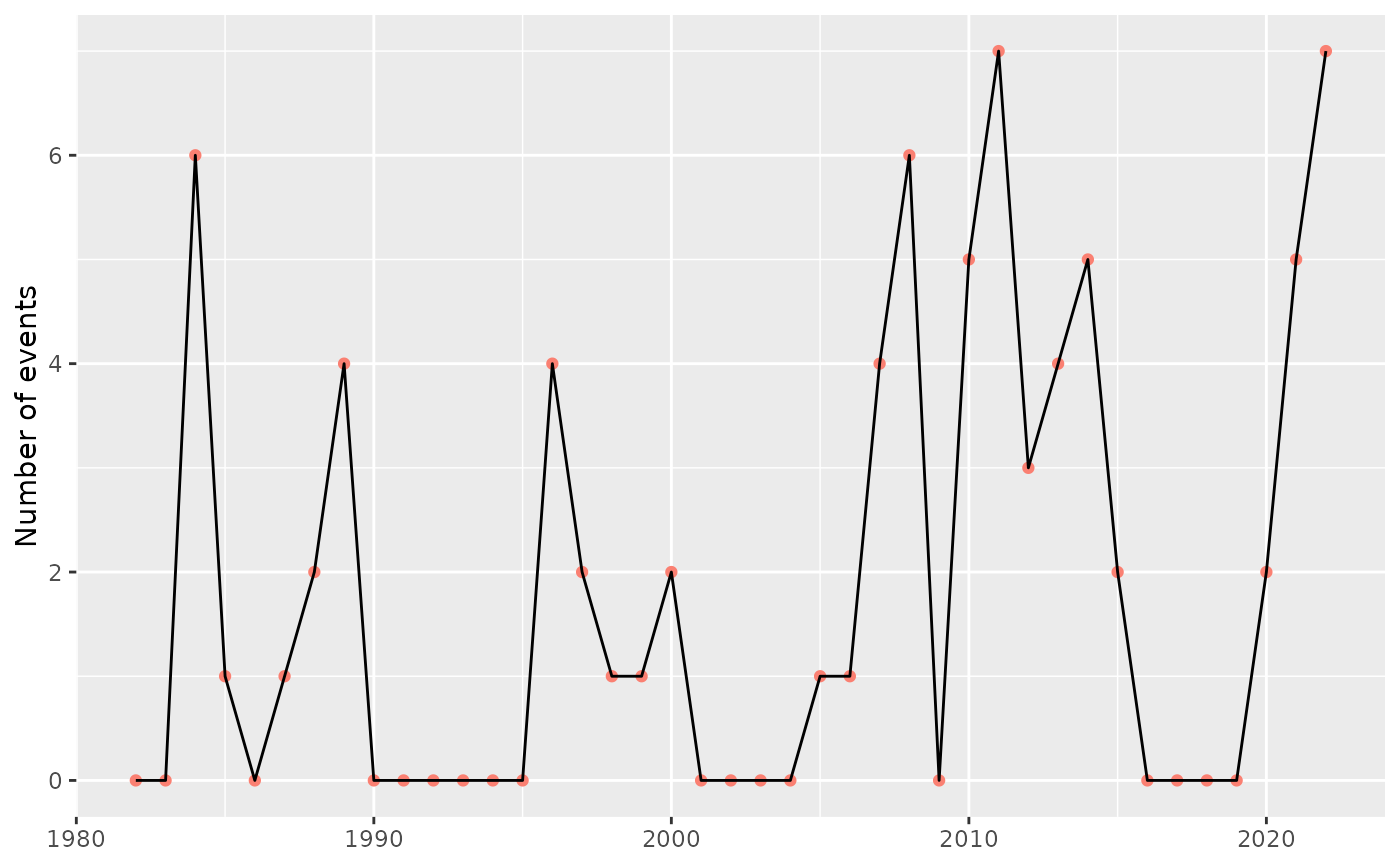Calculate yearly means for event metrics.

block_average(data, x = t, y = temp, report = "full")

## Arguments

data

Accepts the data returned by the detect_event function.

x

This column is expected to contain a vector of dates as per the specification of ts2clm. If a column headed t is present in the dataframe, this argument may be ommitted; otherwise, specify the name of the column with dates here.

y

This is a column containing the measurement variable. If the column name differs from the default (i.e. temp), specify the name here.

report

Specify either full or partial. Selecting full causes the report to contain NAs for any years in which no events were detected (except for count, which will be zero in those years), while partial reports only the years wherein events were detected. The default is full.

## Value

The function will return a data frame of the averaged (or aggregate) metrics. It includes the following:

year

The year over which the metrics were averaged.

count

The number of events per year.

duration

The average duration of events per year [days].

duration_max

The maximum duration of an event in each year [days].

intensity_mean

The average event "mean intensity" in each year [deg. C].

intensity_max

The average event "maximum (peak) intensity" in each year [deg. C].

intensity_max_max

The maximum event "maximum (peak) intensity" in each year [deg. C].

intensity_var

The average event "intensity variability" in each year [deg. C].

intensity_cumulative

The average event "cumulative intensity" in each year [deg. C x days].

rate_onset

Average event onset rate in each year [deg. C / days].

rate_decline

Average event decline rate in each year [deg. C / days].

total_days

Total number of events days in each year [days].

total_icum

Total cumulative intensity over all events in each year [deg. C x days].

intensity_max_relThresh, intensity_mean_relThresh, intensity_var_relThresh, and intensity_cumulative_relThresh

are as above except relative to the threshold (e.g., 90th percentile) rather than the seasonal climatology.

intensity_max_abs, intensity_mean_abs, intensity_var_abs, and intensity_cumulative_abs are as above except as absolute magnitudes rather than relative to the seasonal climatology or threshold.

## Details

This function needs to be provided with the full output from the detect_event or exceedance functions. Note that the yearly averages are calculted only for complete years (i.e. years that start/end part-way through the year at the beginning or end of the original time series are removed from the calculations).

This function differs from the python implementation of the function of the same name (i.e., blockAverage, see https://github.com/ecjoliver/marineHeatWaves) in that we only provide the ability to calculate the average (or aggregate) event metrics in 'blocks' of one year, while the python version allows arbitrary (integer) block sizes.

Note that if this function is used on the output of exceedance, all of the metrics (see below) with relThresh in the name will be returned as NA values.

## Author

Albertus J. Smit, Eric C. J. Oliver, Robert W. Schlegel

## Examples

ts <- ts2clm(sst_WA, climatologyPeriod = c("1983-01-01", "2012-12-31"))
res <- detect_event(ts)
out <- block_average(res)
summary(glm(count ~ year, out, family = "poisson"))
#>
#> Call:
#> glm(formula = count ~ year, family = "poisson", data = out)
#>
#> Deviance Residuals:
#>     Min       1Q   Median       3Q      Max
#> -2.2651  -1.6615  -0.5661   0.7848   3.2940
#>
#> Coefficients:
#>              Estimate Std. Error z value Pr(>|z|)
#> (Intercept) -49.62247   21.43191  -2.315   0.0206 *
#> year          0.02504    0.01069   2.343   0.0191 *
#> ---
#> Signif. codes:  0 ‘***’ 0.001 ‘**’ 0.01 ‘*’ 0.05 ‘.’ 0.1 ‘ ’ 1
#>
#> (Dispersion parameter for poisson family taken to be 1)
#>
#>     Null deviance: 108.10  on 39  degrees of freedom
#> Residual deviance: 102.48  on 38  degrees of freedom
#> AIC: 169.16
#>
#> Number of Fisher Scoring iterations: 6
#>

library(ggplot2)

ggplot(data = out, aes(x = year, y = count)) +
geom_point(colour = "salmon") +
geom_line() +
labs(x = NULL, y = "Number of events")